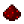# bit.bandFunction bit.band Computes the bitwise AND of two numbers Syntax bit.band(number m, number n) Returns number the value of m AND n Part of ComputerCraft API bit

## ExamplesExample AND the number 18 (10010) with the number 3 (00011), yielding 2 (00010) Code ```print(bit.band(18, 3)) ``` Output 2

## Explanation

All bit operations operate in binary numeral system . An AND operation between two bits yields a 1 if the bits are both 1 and a 0 if either of the bits is 0. This function produces an output by computing the AND of each bit of its two inputs independently. So, for the example above:

 Bit index: Input 1 (18): Input 2 (3): Calculation: Output (2): 4 3 2 1 0 1 0 0 1 0 0 0 0 1 1 3 has a 0 Both 0 Both 0 Both 1 18 has a 0 0 0 0 1 0